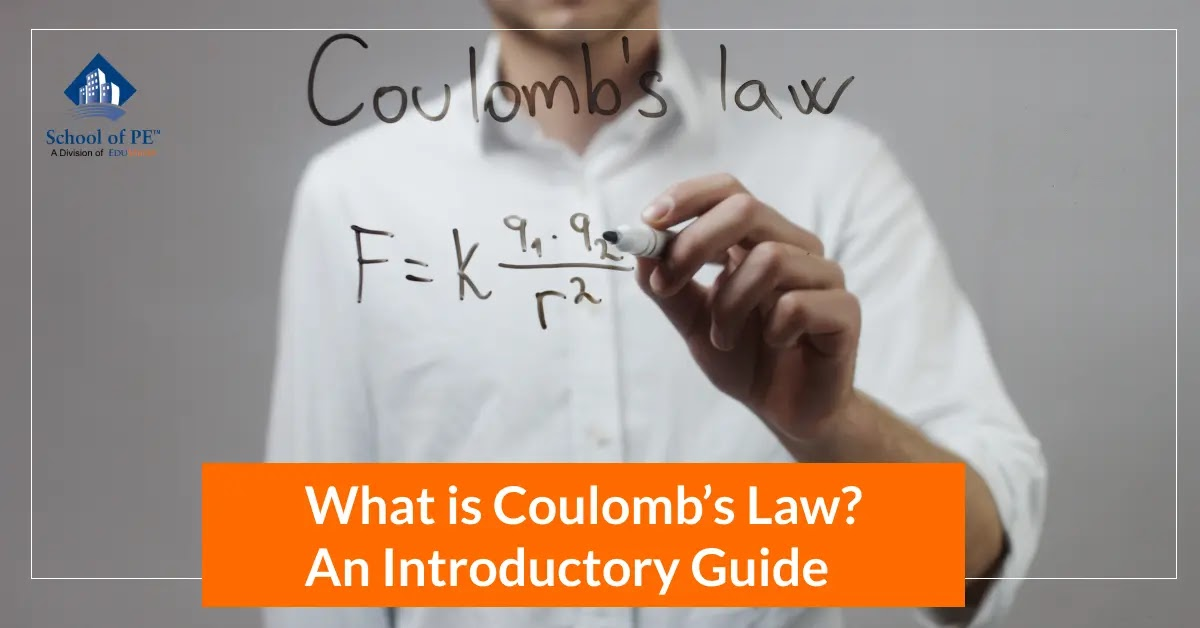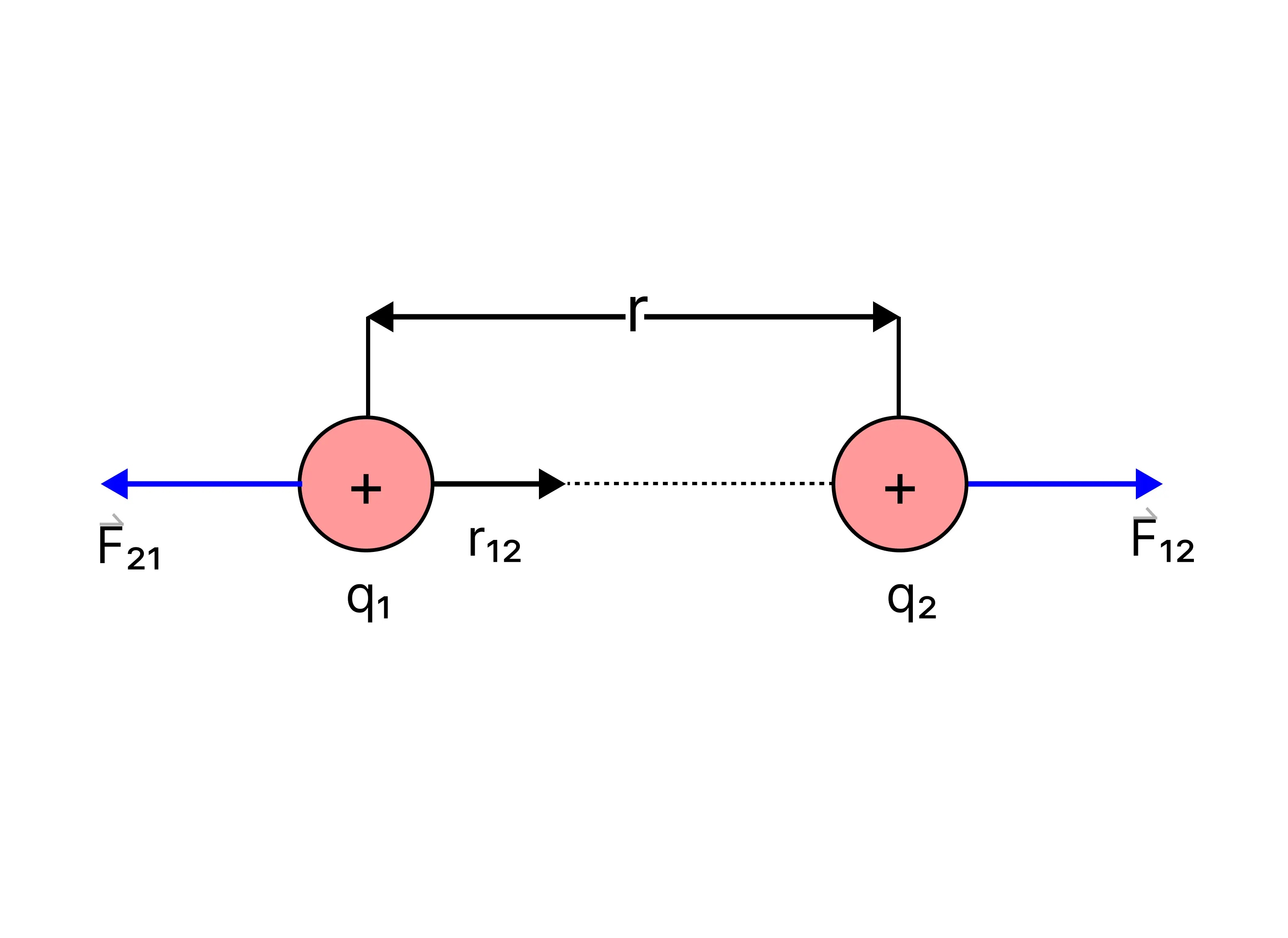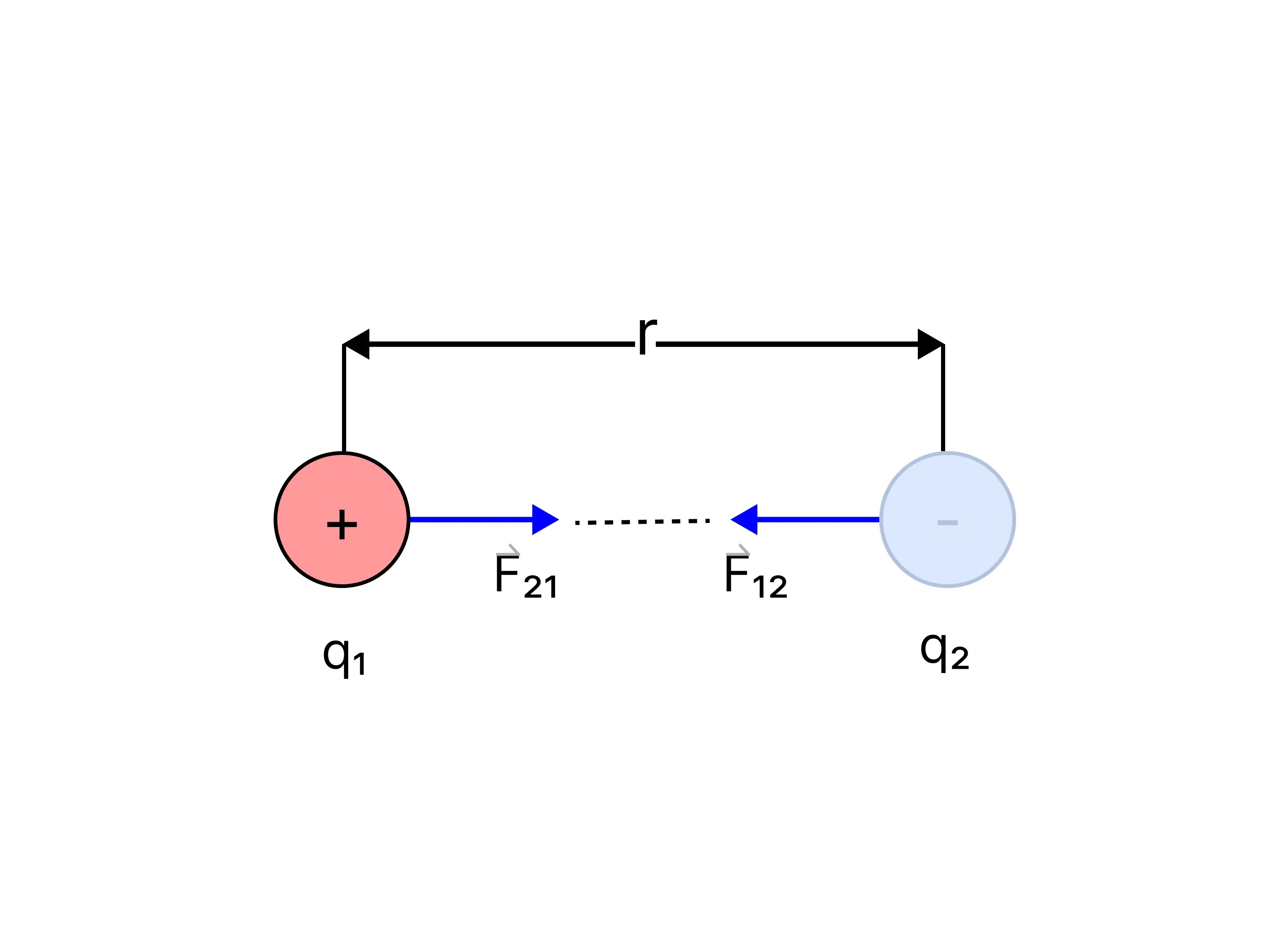Save 31% During Our First Time Ever Cyber Tuesday Sale—Use Promo Code: CYBERTUESDAY Before Midnight. *Restrictions apply.

# What is Coulomb's Law? An Introductory Guide

• 28 July, 2023Introduction

Electricity is all around us, and the study of electromagnetism has led to some of the most significant technological advancements in modern history. One of the fundamental concepts in electromagnetism is Coulomb's law, which describes the force of attraction or repulsion between charged particles. This law was first proposed by French physicist Charles-Augustin de Coulomb in the late 1700s and has been instrumental in our understanding of electricity ever since.

What Is Coulomb's Law?

Charles Coulomb used a device called the torsion balance, which he invented (Figure 1), to measure the electric forces between charged objects. The torsion balance operates on the same principle as the one used by English scientist Henry Cavendish to measure the density of the Earth. In Coulomb's experiment, he replaced the electrically neutral spheres in the apparatus with charged ones, and the resulting electric force between them caused the spheres to either attract or repel one another, twisting the suspended fiber. By measuring the angle of the twist, Coulomb was able to quantify the observed electric force of attraction or repulsion. Since the electric force between the charged spheres is much greater than the force of gravitational attraction, the gravitational force can be ignored (Jewett & Serway, 2013, 694).

Coulomb's law describes the force of attraction or repulsion between two charged particles. The force is directly proportional to the product of the charges and inversely proportional to the square of the distance between them. The Coulomb's law can be expressed in this mathematical term:

Fe = ke |q1 ||q2 |/r2 [N] (1)

where Fe is the electric force between the two charges, q1 and q2 are the charge magnitudes, r is the distance between the charges, and ke is a constant called Coulomb constant.

The International System of Units (SI) unit of ke is

ke = 8.987 x 109 N*m2/C2 (2)

The Coulomb constant can also be expressed in another form:

ke = 1/(4pe0 ) (3)

where the constant e0 is the permittivity of free space, and its value is

e0 = 8.8542 x 10-12 C2/N*m2 (4)

The charge on a proton (+e) or an electron (-e) has the smallest unit of free charge. The values are presented in Table 1.

 Particle Charge (C) Proton (p) +1.60218 x 10-19 Electron (e) -1.60218 x 10-19

Table 1: Charge of Proton and Electron

Attraction and Repulsion of Charge

Coulomb's law deals with force as a vector quantity, meaning it has both magnitude and direction. The electric force exerted by one charged object on another, written as

F?12 = ke |q1 ||q2| / r2 r ^12 (5)

where r ^12 is a unit vector directed from the first charge q1 towards the second charge q2. It is important to remember that the electric force exerted by one charged object on another obeys Newton's third law, meaning the force exerted by q1 on q2 is equal in magnitude and opposite in direction to the force exerted by q1 on q2 . Thus, it can be represented in terms of force, which is .

F?21 = -F?12

Moreover, if q1 and q2 have the same sign, then the product q1q2 is positive, and the electric force on one particle is directed away from the other particle. Figure 2 illustrates the repulsion force between two charges separated by a distance r.Figure 2

On the other hand, if q1 and q2 are of opposite sign, then the product q1q2 is negative, and the electric force on one particle is directed toward the other particle. The attraction force will be illustrated in Figure 3.Figure 3

The signs describe the relative direction of the force, indicating either an attractive or repulsive force. However, the absolute direction of the force on a charge depends on the location of the other charge (Jewett & Serway, 2013, 695-696).

Application of Coulomb's Law

Coulomb's law is a fundamental concept in electromagnetism that has numerous practical applications in our daily lives. For instance, the operation of electronic devices such as cell phones, computers, and televisions is all based on the principles provided by our friend Charles-Augustin de Coulomb. In order to function, these devices rely on the controlled movement of charged particles, electrons, which are governed by Coulomb's Law. The law plays a critical role in determining the behavior of electric charges and electric fields in these devices.

Not only does Coulomb's Law apply to our understanding of electronics, but it also plays a crucial role in understanding the behavior of charged particles in the human body. For instance, the movement of ions across cell membranes is regulated by Coulomb's law. The charged particles in the cell membrane, such as potassium and sodium ions, move in response to electric fields. The interactions between these charged particles play a vital role in the functioning of the human body.

With this fundamental understanding of interactions between charged particles, further scientific research has been made. Some famous examples of applied research are particle accelerators and mass spectrometers, machines that are used in studying the universe. These machines rely on the principles of Coulomb's law to manipulate and control charged particles; particle accelerators, for instance, use electric fields to accelerate charged particles to very high speeds, while mass spectrometers measure the mass-to-charge ratio of ions or charged particles. These marvelous machines are used in experiments to study and quantify the fundamental properties of matter.

Conclusion

To conclude, Coulomb's law is essential in physics and electromagnetism, as it describes the force of attraction or repulsion between charged particles. Recognizing how charged particles interact with one another has allowed numerous real-world applications and has been instrumental in the development of modern technology. By understanding Coulomb's law, we not only have a greater grasp on electronic design, but also on biology and the pursuit of knowledge of our universe.

School of PE's engineering exam review courses have received numerous first-place rankings from third parties. Learn from the best and register for a course today!

References

Jewett, J. W., & Serway, R. A. (2013). Physics for Scientists and Engineers with Modern Physics. Cengage Learning.